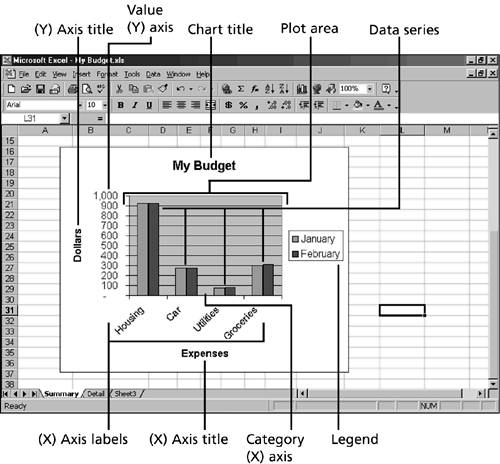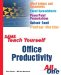# Chart Elements

Before you begin to create charts , you need to be familiar with the chart elements shown in Figure 47.11. Take a few moments to look over the elements of a chart. Figure 47.11 shows a basic column chart with various elements identified.

##### Figure 47.11. Master the chart elements so you can better understand Excel charting.Table 47.2 lists the chart terms and provides an explanation of each chart element that you need to keep in mind when you're working with charts.

##### Table 47.2. Excel's Chart Elements

Element

What It Is

Data series

The bars, pie wedges, or other elements that represent plotted values in a chart. Often, the data series corresponds to rows of data in your worksheet.

X-axis

The number of elements in a series. For most two-dimensional charts, categories are plotted along the Category (X) axis, which is usually horizontal. Categories generally correspond to the columns that you have in your chart data, with the category labels coming from the column headings.

Y-axis

For most two-dimensional charts, data values are plotted along the Value (Y) axis, which is usually vertical. The y-axis reflects the values of the bars, lines, or plot points. In a two-dimensional bar chart, the axes are reversed , with the values being plotted on the x-axis and the categories on the y-axis. In a 3D chart, the z-axis represents the vertical plane, and the x-axis (distance) and y-axis (width) represent the two sides on the floor of the chart.

Legend

The element that designates the separate categories of a chart. For example, the legend for a column chart shows what each column of the chart represents.

Gridlines

The lines that depict the x-axis and y-axis scale of the data series. For example, major gridlines for the y-axis help you follow a point from the x- or y-axis to identify a data point's exact value.Sams Teach Yourself Office Productivity All in One (Sams Teach Yourself All in One)
ISBN: 0672325349
EAN: 2147483647
Year: 2003
Pages: 474
Authors: Greg Perry